# By the graphical method,

Question:

By the graphical method, find whether the following pair of equations are consistent or not. If consistent, solve them.

(i)   3x + y + 4 = 0,6x- 2y + 4 = 0

(ii)  x — 2y — 6, 3x — 6y = 0

(iii) x + y = 3, 3x + 3y =9

Solution:

(I) Given pair of equations is

$3 x+y+4=0$ $\ldots$ (i)

and $6 x-2 y+4=0$ ...(ii)

On comparing with $a x+b y+c=0$, we get

$a_{1}=3, b_{1}=1$

and $c_{1}=4$ [from Eq. (i)]

$a_{2}=6, b_{2}=-2$

and $c_{2}=4$ [from Eq. (ii)]

Here, $\frac{a_{1}}{a_{2}}=\frac{3}{6}=\frac{1}{2} ; \frac{b_{1}}{b_{2}}=\frac{1}{-2}$

and $\frac{c_{1}}{c_{2}}=\frac{4}{4}=\frac{1}{1}$

$\because$ $\frac{a_{1}}{a_{2}} \neq \frac{b_{1}}{b_{2}}$

So, the given pair of linear equations are intersecting at one point, therefore these lines have unique solution.

Hence, given pair of linear equations is consistent.

We have, $3 x+y+4=0$

$\Rightarrow \quad y=-4-3 x$

When $x=0$, then $y=-4$

When $x=-1$, then $y=-1$

When $x=-2$, then $y=2$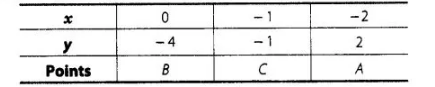and $\quad 6 x-2 y+4=0$

$\Rightarrow \quad 2 y=6 x+4$

$\Rightarrow \quad y=3 x+2$

When $x=0$, then $y=2$

When $x=-1$, then $y=-1$

When $x=1$, then $y=5$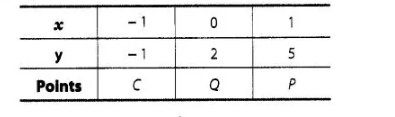Plotting the points $B(0,-4)$ and $A(-2,2)$, we get the straight tine $A B$. Plotting the points $Q(0,2)$ and $P(1,5)$, we get the straight line $P Q$. The lines $A B$ and $P Q$ intersect at $C$ $(-1,-1)$.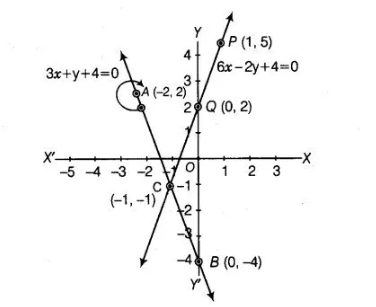(ii) Given pair of equations is $x-2 y=6$ $\ldots$ (i)

and $\quad 3 x-6 y=0$ ...(ii)

On comparing with $a x+b y+c=0$, we get

$a_{1}=\bar{T}, b_{1}=-2$ and $c_{1}=-6 \quad$ [from Eq. (i)]

$a_{2}=3, b_{2}=-6$ and $c_{2}=0$ [from Eq. (ii)]

Here, $\frac{a_{1}}{a_{2}}=\frac{1}{3}, \frac{b_{1}}{b_{2}} \frac{-2}{-6}=\frac{1}{3}$ and $\frac{c_{1}}{c_{2}}=\frac{-6}{0}$

(iii) Given pair of equations is $\quad x+y=3$ $\ldots($ i)

and $3 x+3 y=9$ .....(ii)

On comparing with $a x+b y+c=0$, we get

$a_{1}=1, b_{1}=1$ and $c_{1}=-3$ [from Eq. (i)]

$a_{2}=3, b_{2}=3$ and $c_{2}=-9$ [from Eq. (ii)]

Here, $\frac{a_{1}}{a_{2}}=\frac{1}{3}, \frac{b_{1}}{b_{2}}=\frac{1}{3}$ and $\frac{c_{1}}{c_{2}}=\frac{-3}{-9}=\frac{1}{3}$

$\Rightarrow$ $\frac{a_{1}}{a_{2}}=\frac{b_{1}}{b_{2}}=\frac{c_{1}}{c_{2}}$

So, the given pair of lines is coincident. Therefore, these lines have infinitely many solutions. Hence, the given pair of linear equations is consistent.

Now, $x+y=3 \Rightarrow y=3-x$

If $x=0$, then $y=3$. If $x=3$, then $y=0$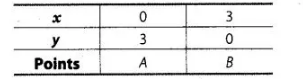and $3 x+3 y=9 \Rightarrow 3 y=9-3 x$

$\Rightarrow$ $y=\frac{9-3 x}{3}$

If $x=0$, then $y=3$; if $x=1$, then $y=2$ and if $x=3$, then $y=0$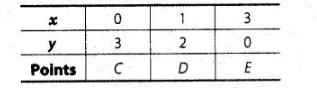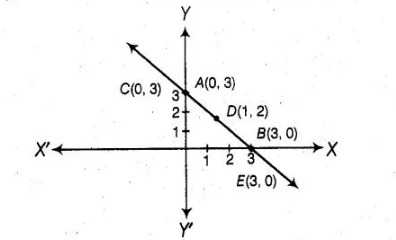Plotting the points $A(0,3)$ and $B(3,0)$, we get the line $A B$. Again, plotting the points $C(0,3) D(1,2)$ and $E(3,0)$, we get the line $C D E$.

We observe that the lines represented by Eqs. (i) and (ii) are coincident.